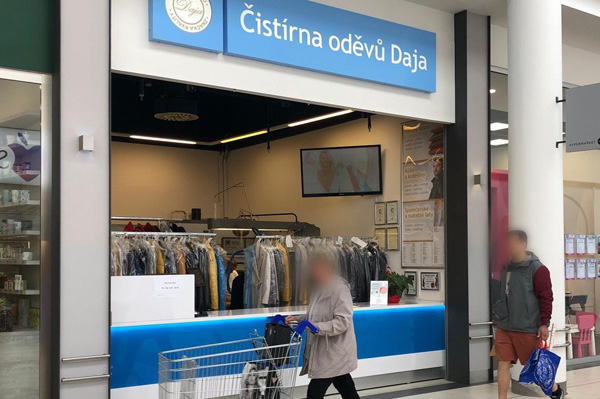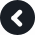Accept

Telephone: +420 495 260 829

Opening hours:
Mon - Sun 8:00 am - 8:00 pm

How to get to us:
Autobus: 1,2,27,28
Parking available on sitex x x x x x x x x x x x x x x x x x x x x x x x x x x x x x x x x x x x x x x x x x x x x x x x x x x x x x x x x x x x x x x x x x x x x x x x x x x x x x x x x x x x x x x x

## Our Awards